Welcome to Study Material Solution
Leave Comment

## INTRODUCTION

· Electricity can be represent by the charge. If body is charged by friction and the charge is stationary then the electricity is called frictional electricity.
· If charge is moving from one place to another through the conductor it is called current electricity.

## ELECTRICITY CIRCUITS

· The path of flow of electricity from one terminal of the cell and returning to the other is called and electrical circuit.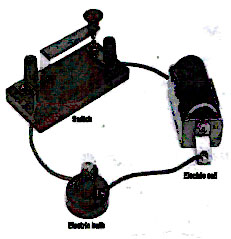(a) Open Electric Circuit : An electric circuit through which no electric current flows is known as open electric circuit. The electric
circuit or it the connecting wires break from any point.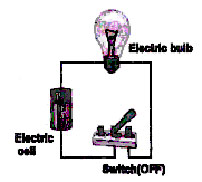(b) Closed Circuit : An electric circuit through which electric current flows continuously is known as closed circuit.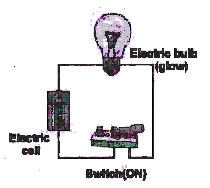· For flow of electricity, the circuit must be made of conductors, insulators in the path of electrical circuits makes the circuit incomplete.

ELECTRICAL CELLS

Electrical cells are the sources of electric current.
Types of electrical cell : (i) primary
(ii) secondary

(i) Primary Cells :      The cells which cannot be charged again and again are known as primary cells.
Eg : Voltaic, Daniel, laclanche and dry cells.

(ii) Secondary Cells : The cells which can be charged again and again are known as secondary cells.
Eg : Edison cell, lead - acid accumulator.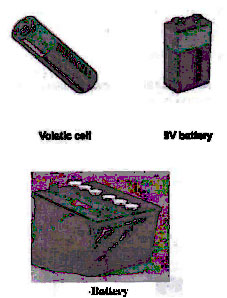Note : Combination of cell is known as battery.

CIRCUIT DIAGRAM AND CIRCUIT SYMBOLS

Scientists use certain symbols to draw electrical circuits. An electrical circuit drawn using these symbols is called a circuit diagram.
The symbols used to indicate different components are called circuit symbols. Figure shows some components and their circuit symbols.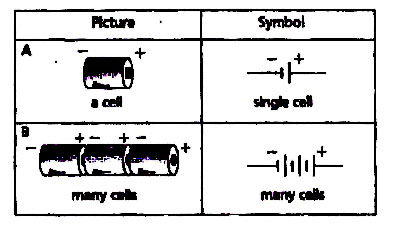An electrical circuit in symbols :REQUIREMENTS OF A CIRCUIT
Suppose that you were given a small light bulb, an electrochemical cell and a bare copper wire and were asked to find
the four different arrangements of the three items that would
result in the formation of an electric circuit that would light the bulb.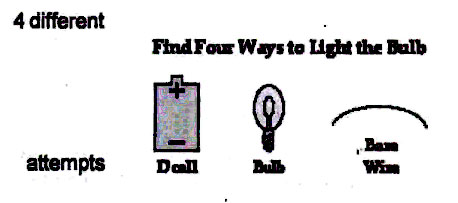In attempt A, the wire does not loop back to the negative terminal of the cell. In attempt 8, the wire does form a loop
but not back to the negative terminal of the cell. In attempt C,there is no complete loop at all. Attempt D resembles attempt
B in that there is a loop but not from the positive terminal to the negative terminal. And in attempt E, there is a loop and
it does go from positive terminal to negative terminal; this is a circuit but the light bulb is not included as part of it. CAUTION:
Attempt E will cause your fingers to get hot as you hold the bare wire and charge begins to flow at a high rate between the positive and negative terminals.### LIGHT BULB ANATOMY

A light bulb is a relatively simple device consisting of a filament resting upon or somehow attached to two wires. The wires and the filament are
conducting materials that allow charge toflow through them. One wire is connected to the ribbed sides of the light bulbs. The other wire is connected
to the bottom base of the light bulb. The ribbed edge and the bottom base are separatedby an insulating material that prevents the direct flow of charge
between the bottom base and the ribbed edge. The only pathway by which charge can make it from the ribbed edge to the bottom baseor vice versa is
the pathway that includes the wires and the filament. Charge can either enter the ribbed edge, make the pathway through the filament and exit out the
bottom base; or it can enter thebottom base, make the pathway through the filament and exit out the ribbed edge. As such, there are two possible entry
points and two corresponding exit points.

The successful means of lighting the bulb as shown above involved placing the bottom base of the bulb on the positive terminal
and connecting the ribbed edge to the negative terminal usinga wire. Any charge that enters the light bulb at the bottom base exits
the bulb at the location where the wire makes contact with the ribbed edge. The second arrangement that lead to a lit light bulb
involve placing the bulb at the negative terminal of the cell. A wire must then connect the other part of the bulb to the positive terminal of the cell.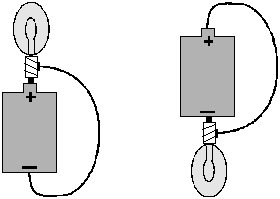Successful attempts at lighting the electric bulb.

### CONDUCTORS AND INSULATORS

(i) Conductors :  Those substances through which electric charges can flow, are called conductors.
Eg : silver, copper and aluminium etc.

(ii) Insulators : The material in which there is no flow of current are called insulators.
Eg: Plastic, rubber and wood etc.

### The requirement of a closed conducting path

There must be a closed conducting path that extends from the positive terminal to the negative terminal. 11 is not enough that there is simply a closed
conducting loop; the loop itself must extend fromthe positive terminal to the negative terminal of the electrochemical cell. An electric circuit is like a
water circuit at a water park. The flow of charge through wires is similar to the flow of water through the
pipes and along the slides at a water park. If a pipe gets plugged or broken such that water cannot make the complete path through the circuit, then the
flow of water will soon cease, In an electric circuit,all connections must be made and made by conducting materials capable of carrying charge. As the cell,
\ bulb and wire experiment continues, some students explore the capability of various materials to
carry a charge by Inserting them in their circuit. Metallic materials are conductors and can be inserted into the circuit to successfully light the bulb. On the other hand, paper and plastic materials are typically
insulators and their insertion within the circuit will hinder the flow of charge to such a degree that the current ceases and the bulb no longer lights. There must be a closed conducting loop from the positive to
the negative terminal in order to establish a circuit and to have a current.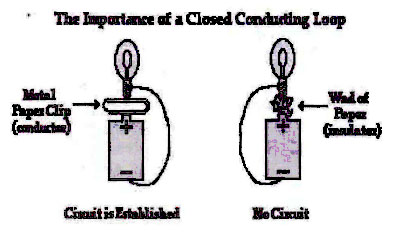#### OBJECTIVE QUESTIONS

1. The switch is in the OFF state. It means that
(A) the circuit is open .
(B) the circuit is closed.
(C) there is no key in the circuit.
(D) there is no battery in the circuit

2. Which is the best conductor?
(A) Carbon       (B) Copper
(C) Iron            (D) Aluminium

3. Materials which allow larger current to flow through them are called :
(A) Insulators     (B) Semi-conductors
(C) Alloys          (D) Conductors

4. Bakelite Is a/an :
(A) semi conductor            (B) conductor
(C) insulator                      (D) None of the above

5. Examples of primary cells are :
(A) Voltaic                          (B) Daniel
(C) Dry cell                        (D) All of them

6. Electro-chemical cell used in torches is :
(A) Voltaic cell                  (B) Daniel cell
(C) Fuel cell                      (D) Leclanche dry cell

7. Which of the following is not the advantage of dry cell?

(A) It is compact and convenient to use
(B) It can be recharged
(C) It is handy to carry
(D) None of them

8. Which of the following is an example of secondary cell :

(A) Voltaic cell
(B) Daniel cell
(D) Dry cell

9. While an electrician repairs an electric switch he wears rubber gloves to

(A) just for fun
(B) save electricity
(C) prevent him for shock
(D) All of these

10. The combination of cell in proper order is called-
(A) mega cell               (B) battery
(C) giga cell                 (D) None of these

11. A device which is used to stop supply of current in the circuit is called -
(A) battery                  (B) circuit
(C) wire                      (D) switch

12. A substance that does not allow the current to flow through it is called -
(A) conductor             (B) insulator
(C) semiconductor     (D) superconductor

13. Iron is
(A) Conductor           (B) Insulator
(C) semiconductor    (D) None of these

14. Electrical wires are covered with

(A) insulating material
(B) conducting material
(C) semi - insulator material
(D) All of these

15. How many terminals are there in a battery?
(A) 3                        (B) 2
(C) 1                        (D) 4

16. A filament of low melting point is used in
(A) electric bulb      (B) wire
(C) fuse                   (D) electric iron

SUBJECTIVE QUESTIONS

1. Explain the following terms :

(a) electrical circuit
(b) circuit symbols
(c) primary and secondary cells
(d) volt
(e) watt.

2. What are the conditions for electricity to flow in an electrical circuit?

3. Distinguish between the conductors and insulators.

4. What is the open and closed circuit?

5. What is LED? State its use in our daily life.

1. A        2. B       3. D        4. C         5. D         6. B       7. B          8. C          9. C           10. B            11. D           12. B           13. A                14. A             15. B             16. C

QUESTIONS

1. If I is the current through a wire and e is the charge of electron, then number of electrons in t seconds will be

(a)Ie/t           (b) Ite            (c) e/It             (d) It/e

2. The unit for electric conductivity is

(a) ohm per cm        (b) ohm ×cm         (c) ohm per second         (d) mho

3. The direction of flow of current is (conventionally) :

(a) The direction of flow of electrons
(b) The direction opposite to the flow of electrons
(c) Sometimes in the direction of the flow of electrons and sometime opposite to it
(d) None of these

4. A free electron :

(a) moves from a higher potential region to a lower potential region
(b) moves from a lower potential region to a higher potential region
(c) does not move at all
(d) none of the above

5. If there is no flow of charge between two charged bodies when they are connected to each other :

(a) they have the same quantity of charge                (b) they have the same capacity
(c) they have the same potential                               (d) none of the above

6. If a charged body attracts another body, then charge on the other body
(a) must be negative                                                (b) must be positive
(c) must be zero                                                       (d) may be negative or positive or zero

7. Conventionally, the direction of the current is taken as the direction of flow

(a) to negative charge
(b) the direction of flow of atoms
(c) of molecules
(d) of positive charge

8. Electric potential is :

(a) a scalar quantity
(b) a vector quantity
(c) neither a scalar quantity nor a vector quantity
(d) in some cases, scalar and in some cases, vector

9. Which of the following terms does not represent electrical power in a circuit?
(a) I2R            (b) IR2                (c)VI              (d)

10. A electric bulb is rated 220 V and 100 W. When it is operated on 110 V, the power consumed will be :
(a) 100 W      (b) 75 W             (c) 50 W         (d) 25 W

11. What is the potential difference across the source ?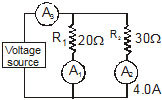(a) 440 V     (b) 220 V            (c) 120V          (d) 60V

12. If 10 cells each of 1.4 volts are connected in parallel, the equivalent potential difference will be
(a) 1.4 volts  (b) 0.14 volts     (c) 14 volts     (d) 10/.4 volts

13. Joule/coulomb is equivalent to :
(a) Watt        (b) Ampere        (c) Volt           (d) Ohm

14. A voltmeter is used for measuring :
(a) Electric current                      (b) Resistance
(c) Potential difference               (d) Electric Power

15. Three charges –q, Q and –q are placed at equal distance on a straight line. If the total potential energy of the system of three charges is zero, then then the ratio Q :  q is
(a) 1 : 2               (b) 2 : 1              (c) 1 : 1              (d) 1 : 4

16. Particle A has a charge +q and particle B has a charge +4q, each having the same mass m. When allowed to fall from rest through the same potential difference, the ratio of their speeds vA/vB will be-
(a) 2 : 1              (b) 1 : 2               (c) 1 : 4              (d) 4 : 1

17. An electric charge on a body produces
(a) a magnetic field only
(b) an electric field only
(c) both electric and magnetic field
(d) neither electric nor magnetic field

18. A suitable unit for expressing the strength of electric field is
(a) V/C                                     (b) C/m
(c) N/C                                     (d) C/N

19. 20 coulomb charge is flowing in 0.5 second from a point in an electric circuit then value of electric current in amperes will be
(a) 10                                      (b) 40
(c) 0.005                                 (d) 0.05

20. When a fuse is rated 8 A, it means

(a) it will not work if current is less than 8 A
(b) it has a resistance of 8 ohm
(c) it will work only if current is 8 A
(d) it will burn if current exceeds 8 A

21. Cost of electricity for home use is Rs. 1.50 per unit. This unit is

(a) 1 ampere                         (b) 1 volt
(c) 1 joule                             (d) 1 kilowatt hour

22. If one micro-amp. current is flowing in a wire, the number of electrons which pass from one end of the wire to the other end in one second is

(a) 6.25×1012                       (b) 6.25×1015
(c) 6.25×1018                       (d) 6.25×1019

23. The no. of electrons flowing per second through any cross section of wire, if it carries a current of one ampere, will be

(a) 2.5×1018                        (b) 6.25×1018
(c) 12.5×1018                      (d) 5×1018

24. The total momenturm of electrons in a straight wire of length l = 1000 m carrying a current I = 70 A , will be (in N. s)

(a) 0.40×10–6                     (b) 0.20×10–6
(c) 0.80×10–6                    (d) 0.16×10–6

25. When there is an electric current through a conducting wire along its length then an electric field must exist

(a) inside the wire but normal to it
(b) inside the wire but parallel to it
(c) outside the wire but normal to it
(d) outside the wire but parallel to it

26. Three wires of resistance 18W, 15W & 10W are connected in parallel. The current in the 18W wire is 0.75A. What is the current in the 15W wire ?
(a) 0.6 A                              (b) 0.9 A
(c) 1.2 A                              (d) 1.8 A

27. What is the total current supplied by the battery to the circuit shown in the adjoining figure ?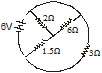(a) 1 A                               (b) 2 A
(c) 4 A                               (d) 6 A

28. What is the current through a 5.0 ohm resistor if the voltage across it is 10 V

(a) Zero                           (b) 0.50 A
(c) 2.0 A                          (d) 5.0 A

29. 2 ampere current is flowing through a conductor from a 10 volt emf source then resistance of conductor is

(a) 20ohm                      (b)5 ohm
(c)12ohm                       (d)8ohm

30. The slope of voltage (V) versus current (I) is called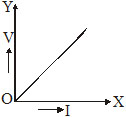(a) resistance                 (b) conductance
(c) resistivity                 (d) conductivity

31. Kilowatt-hour = :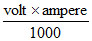(a) Time in seconds      (b) Time in hours
(c) Time in minutes      (d) Time in days

32. If the length of the wire is doubled and its cross-section is also doubled, the resistance will :

(a) increase four times
(b) decrease four times
(c) increase eight times
(d) remains unchanged

33. The resultant resistance of a circuit connecting resistors is parallel is :

(a) Equal to the sum of the individual resistors
(b) Smaller than the smallest resistance
(c) Greater than the largest resistance
(d) Can’t say

34. When two resistance R1 and R2 are connected in parallel their equivalent resistance is :

(a) R1 + R2
(b)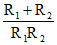(c)(d)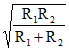35. The length of a wire is doubled and the radius is doubled. By what factor does the resistance change

(a) 4 times as large                  (b) twice as large
(c) unchanged                         (d) half as large

36. You are given n identical wires, each of resistance R. When these are connected in parallel, the equivalent resistance is X. When these will be connected in series, then the equivalent resistance will be
(a) X/n2                                   (b) n2X
(c) X/n                                     (d) nX

37. Three resistor of and are connected in series. What is their equivalent resistance
(a)                                             (b)
(c)                                             (d)

38. A wire of resistance R is cut into ten equal parts which are then joined in parallel. The new resistance is

(a) 0.01 R                               (b) 0.1 R
(c) 10 R                                  (d) 100 R

39. If a wire stretched to  make its length three times, its resistance will become
(a) three times                       (b) one-third
(c) nine times                        (d) one-ninth

40. A wire of resistance 12 ohms is bent in the form of a circular ring. The effective resistance between the two points on any diameter of the circle is
(a) 24 ohm                            (b) 12 ohm
(c) 6ohm                               (d) 3 ohm

41. Two wires of resistance R1 and R2 are joined in parallel. The equivalent resistance of the combination is

(a) R1R2/R1 + R2               (b)  R1 + R2
(c) R1 × R2                         (d) R1 / R2

42. The primary cell which is used in daily life is
(a) Leclanche cell               (b) Dry cell
(c) Daniel cell                     (d) Simple voltaic cell

43. Which one of the following primary cells has emf. 1.08 volts and which remains fairly constant
(a) Daniel cell                    (b) Simple voltaic cell
(c) Leclanche cell              (d) Dry cell

44. Primary cell are connected in parallel to

(a) Increase voltage
(b) decrease capacity
(c ) decrease internal resistance
(d) make electric current constant

45. In a circuit, a voltmeter is always connected in :

(a) Parallel                      (b) Series
(c) Parallel or Series       (d) Can’t say

46. If the voltage applied to an immersion heater is doubled, the rate of rise of the temperature of water will approximately by

(a) same                         (b) double
(c) half                           (d) four times

47. The existence of a negative charge on a body implies that it has :

(a) Lost some of its electron
(b) Lost some of its protons
(c) Acquired some electrons from outside
(d) Acquired some protons from outside

48. What is the current through a 5.0 ohm resistor if the voltage across it is 10 V :

(a) zero                         (b) 0.50 A
(c) 2.0 A                        (d) 5.0 A

49. What is immaterial for an electric  fuse wire :

(a) Its specific resistance
(c) Its length
(d) Current flowing throught it

50. A 1500 watt heater is connected to a 120 volt source for 2.0 h. How much heat energy is produced :

(a) 1.1 × 107 J                 (b) 1.8 × 105 J
(c) 9.0 × 104 J                 (d) 3.0 × 103 J